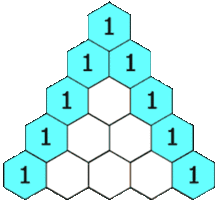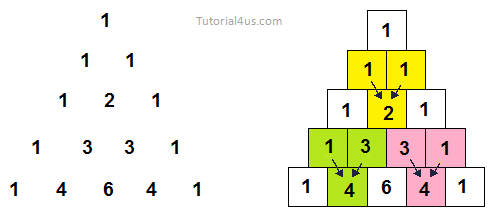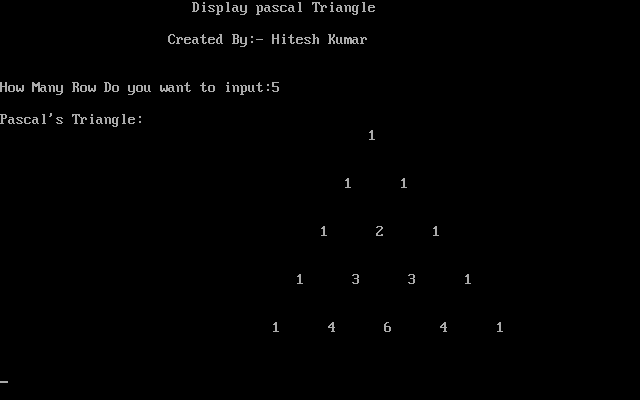# Print Pascal Triangle in C

## Program to Print Pascal Triangle in C

The concept of pascal triangle is; Pascal's triangle is a set of numbers arranged in the form of a triangle. Each number in a row is the sum of the left number and right number on the above row. If a number is missing in the above row, it is assumed to be 0. The first row starts with number 1. The following is a Pascal triangle with 5 rows.

Pascal's triangle is a triangular array of the binomial coefficients.## Program to Print Pascal Triangle in C

```#include<stdio.h>
#include<conio.h>

void main()
{
int bin,p,q,r,x;
clrscr();
bin=1;
q=0;
printf("\t\t\tDisplay pascal Triangle");
printf("\n\n\t\t     Created By:- Hitesh Kumar");
printf("\n\n\nHow Many Row Do you want to input:");
scanf("%d",&r);

printf("\nPascal's Triangle:\n");

while(q<r)
{
for(p=40-3*q;p>0;--p)
printf(" ");
for(x=0;x<=q;++x)
{
if((x==0)||(q==0))
bin=1;
else
bin=(bin*(q-x+1))/x;
printf("      ");
printf("%d",bin);
}

printf("\n\n\n");
++q;
}
getch();
}
```

### OutputWIFI CCTV Camera